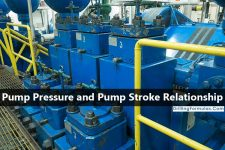## Pump Pressure and Pump Stroke RelationshipThere are relationships between pump pressure and pump stroke that you really need to understand and be able to determine pump pressure after adjusting new pump stroke.

There are 2 formulas used to determine pump pressure as shown in the detail below:

## 1st formula for estimating new circulating pressure (simple and handy for field use)

New circulating pressure in psi = present circulating pressure in psi x (new pump rate in spm ÷ old pump rate in spm) 2

Example: Determine the new circulating pressure, psi using the following data:
Present circulating pressure = 2500 psi
Old pump rate = 40 spm
New pump rate = 25 spm
New circulating pressure in psi = 2500 psi x (25 spm ÷ 40 spm) 2
New circulating pressure = 976.6 psi

## 2nd formula for estimating new circulating pressure (more complex)

For the 1st formula, the factor “2” is used but it’s just the round up figure. If you want more accurate figure, you need to figure out an exact figure. So the 2nd formula has one additional formula to calculate the factor based on 2 pressure readings at different pump rate.  Please follow these steps to determine new circulating pressure

1. Determine the factor ”n” and  the formula to determine factor “n” is below:

Factor (n) = log (pressure 1 ÷ pressure 2) ÷ log (pump rate 1÷pump rate 2)

2. Determine new circulating pressure with this following formula.

New circulating pressure in psi = present circulating pressure in psi x (new pump rate in spm ÷ old pump rate in spm) n

Note: factor “n” comes from the first step of calculation.

Example: Determine the factor “n” from 2 pump pressure reading
Pressure 1 = 2700 psi at 320 gpm
Pressure 2 = 500 psi at 130 gpm
Factor (n)   = log (2700 psi ÷ 500 psi) ÷ log (320 gpm ÷ 130 gpm)
Factor (n) = 1.872

Example: Determine new circulating pressure by using these following information and the factor “n” from above example:
Present circulating pressure = 2500 psi
Old pump rate = 40 spm
New pump rate = 25 spm
New circulating pressure, psi = 2500 psi x (25 spm ÷ 40 spm) 1.872
New circulating pressure = 1037 psi

Ref books: Lapeyrouse, N.J., 2002. Formulas and calculations for drilling, production and workover, Boston: Gulf Professional publishing.

Bourgoyne, A.J.T., Chenevert , M.E. & Millheim, K.K., 1986. SPE Textbook Series, Volume 2: Applied Drilling Engineering, Society of Petroleum Engineers.

Mitchell, R.F., Miska, S. & Aadny, B.S., 2011. Fundamentals of drilling engineering, Richardson, TX: Society of Petroleum Engineers.